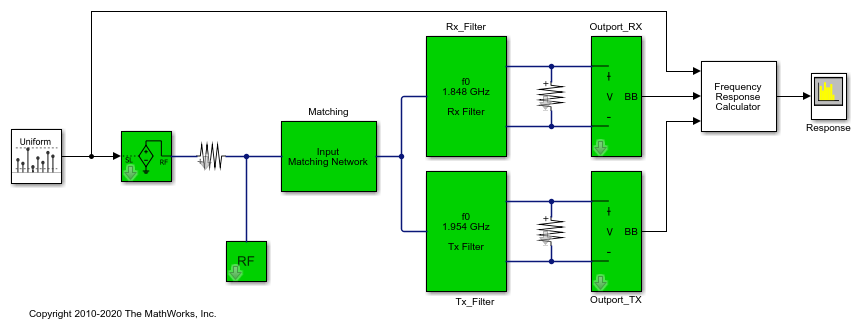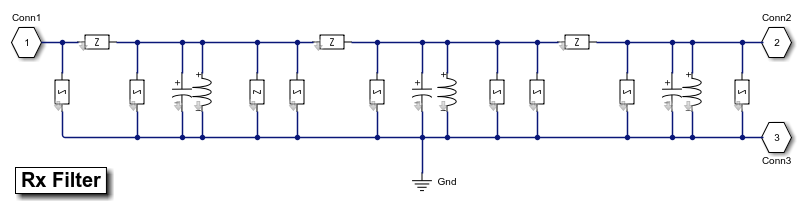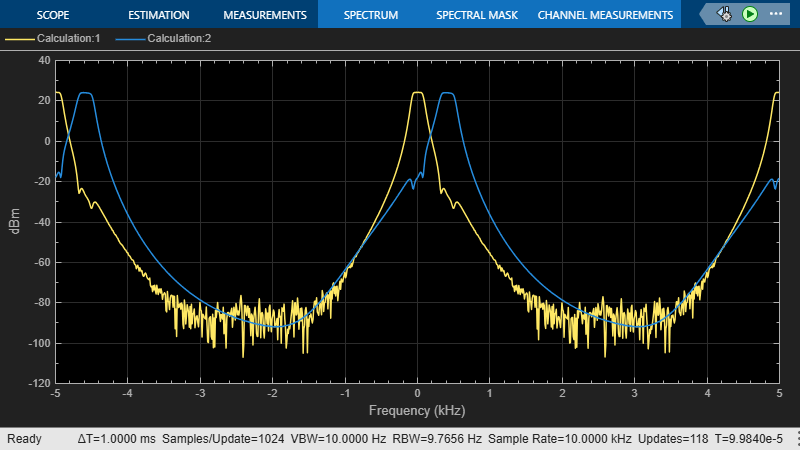# Frequency Response of an RF Transmit/Receive Duplex Filter

This example shows how to use blocks from the RF Blockset™ Circuit Envelope library to simulate a transmit/receive duplex filter and calculate frequency response curves from a broadband white-noise input. Blocks from the DSP System Toolbox™ libraries generate the input signal, process the output signal, and display the results.### System Architecture

The system consists of:

• A DSP System Toolbox Random Source block, which generates broadband white noise.

• Inport and Resistor blocks from the RF Blockset circuit envelope library, which model a controlled voltage source with internal impedance.

• An input matching network, which maximizes the power of the signal received by the filters.

• Duplexed transmit and receive filters, composed of circuit envelope blocks from the RF Blockset Elements sublibrary. The center frequencies of the filters are 1.954 GHz and 1.848 GHz, and the bandwidths are 27 MHz and 18 MHz, respectively. The receive filter is shown in the figure below.

• Two Outports acting as voltage sensors, measuring the voltage across two load resistors.

• The Frequency Response Calculator subsystem, which computes the voltage transfer functions.

• A DSP System Toolbox Spectrum Analyzer, which displays the frequency response curves.

Block parameters in this example are specified by variables stored in the model workspace. To alter the model workspace, select Modeling > Model Explorer and click on the Model Workspace node for this model in the Model Hierarchy pane.### Running the Example

1. Type `open_system('simrfV2_duplexer')` at the Command Window prompt.

2. Select Simulation > Run.

The Spectrum Analyzer displays the envelope voltage transfer functions of the two filters. Duplexing is evident in the frequency response of the two filters. The frequency values along the-axis are relative to the RF carrier. To compute the absolute frequencies, add the value of the Carrier frequencies parameter of the Inport block to the frequencies shown.### Calculating a Transfer Function Using a Broadband White Noise Source

The Frequency Response Calculator subsystem processes the Simulink® signals converted from the RF Blockset environment at the Outport blocks. Within this subsystem, two Compute Transfer Function subsystems correlate the outputs to the input signal.

The Compute Transfer Function subsystems operate on discrete signals. To configure the RF Blockset circuit envelope environment for discrete-time simulation:

• In the Configuration block dialog, select `Auto` from the Solver drop-down menu, and specify the Step size parameter. Within the RF Blockset circuit envelope environment, the local solver overrides the solver options specified in the Configuration Parameters dialog box.

• Use a continuous source, or use a discrete source with a sample time equal to the value specified in the Configuration block. In this example, the Sample mode parameter of the Random Source block is `Continuous`.